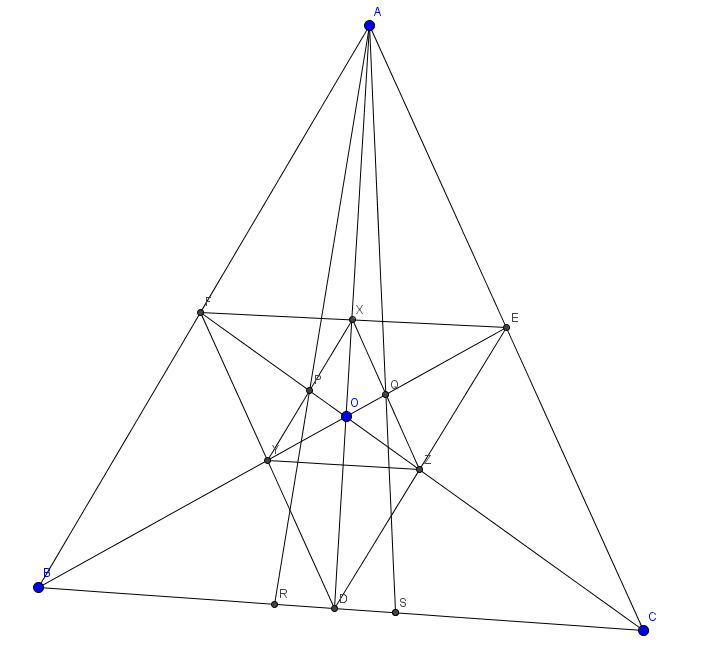# The Special Point In A Triangle 1

Geometry Level 5Let $O$ be a point in triangle $ABC$.

$D$ is the intersection of $AO$ and $BC$. $E,F$ are defined similarly.

$X$ is the intersection of $EF$ and $AD$. $Y,Z$ are defined similarly.

Let $P$ be the intersection of $XY$ and $CF$ and $Q$ be the intersection of $XZ$ and $BE$.

$R$ is the intersection of $AP$ with $BC$ and $S$ is the intersection of $AQ$ with $BC$.

Find: $\dfrac{4 \times BS \times RC}{SC \times BR}$.

The Special Point in A Triangle 2

×